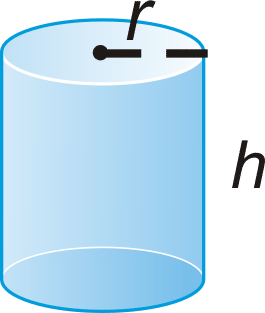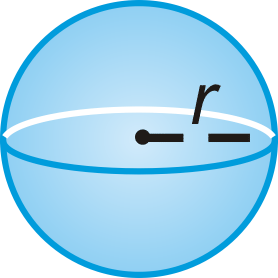# 2.4.8: Solving for a Variable

•• Contributed by CK12
• CK12

## Solving Algebraic Equations for a Variable

You are planning a trip to Spain in the summer. In the U.S., we use Fahrenheit to measure the temperature, but in Europe, they use Celsius. To get yourself used to Celsius, you find the conversion equation F=(9/5)C+32. You can substitute the temperature in Celsius for C and solve the equation for the temperature in Fahrenheit, F. When you start looking at the weather in Spain in the summer, the website says it is usually 35∘C. What will the temperature be in Fahrenheit?

### Solving for a Variable in an Algebraic Equation

Solving an algebraic equation for a variable can be tricky, but it can also be very useful. This technique can be used to go back and forth between two different units of measurement. To solve for, to isolate, a variable within an equation, you must undo the operations that are in the equation.

Let's solve each equation for the specified variable.

1. Solve for x in the equation 3x−4y=12.

To solve for x, you need to move the 4y to the other side of the equation. In order to do this, we must do the opposite operation. Since the 4y is being subtracted, we must add it to the other side of the equation.

3x−4y=12 +4y=+4y

3x=4y+12

Now we need to get x alone. 3x is the same as “3 multiplied by x.” Therefore, to undo the multiplication we must divide both sides of the equation by 3.

3x/3=(4y/3)+12/3

x=(4/3)y+4

When undoing multiplication, you must divide everything in the equation by 3.

A few things to note:

• To undo an operation within an equation, do the opposite.
• Perform the opposite operations in the reverse order of the Order of Operations.
• Combine all like terms on the same side of the equals sign before doing the first bullet.
1. Given the equation (2b/3)+6a−4=8, find b when a=1 and a=−2.

First, isolate b. Move both the 6a and the 4 over to the other side by doing the opposite operation.

(2b/3)+6a−4=8 −6a+4=+4−6a

(2b/3)=12−6a

Now, we have a fraction multiplied by b. To undo this we must multiply by the reciprocal of 2/3, which is 3/2. This means, we must multiply everything in the equation by the reciprocal.

2b/3=12−6a

3/2(2b3=12−6a)

(3/2)⋅(2b/3)=(3/2)⋅12−(3/2)⋅6a

b=18−9a

Even though we know that a 9 can be pulled out of the 18 and the 9 in the above equation, it is not a necessary step in solving for b. Now, we can do what the question asks, find b when a=1 and -2. Plug in these values for a.

a=1: b=18−9(1)=18−9=9

a=−2: 18−9(−2)=18+18=36

1. The area of a triangle is A=(1/2)bh, where b is the base of the triangle and h is the height. You know that the area of a triangle is 60 in2 and the base is 12 in. Find the height.

First solve the equation for h.

A=(1/2)bh

2⋅A=2⋅(1/2)bh Multiply both sides by 2.

2A=bh Divide both sides by b.

(2A/b)=h

Now, plug in what we know to find h. 2(60)/12=120/12=10. The height is 10 inches.

This process is helpful when solving for any variable in any equation or formula. Some formulas you may need to know for this text are listed below.

Distance d=rt d=distance,r=rate,t=time
Temperature F=95C+32 F=degrees in Fahrenheit,C=degrees in Celsius
Area of a Triangle A=12bh A=area,b=base,h=height
Area of a Rectangle A=bh A=area,b=base,h=height
Area of a Circle A=πr2 A=area,r=radius
Area of a Trapezoid A=12h(b1+b2) A=area,h=height,b1=one base,b2=other base
Perimeter of a Rectangle P=2l+2w P=perimeter,l=length,w=width
Circumference of a Circle C=2πr C=circumference,r=radius

### Examples

Example 2.4.8.1

Earlier, you were asked to convert Celsius into Fahrenheit.

Solution

First, let's substitute in C=35∘ to find the degrees in Fahrenheit.

F=95⋅35+32

F=63+32

F=95

As you can see, a good estimator for the conversion is to double the degrees, when in Celsius, and then add 32. Needless to say, it is going to be quite hot in Spain and you should pack summer clothes for your trip.

Example 2.4.8.2

Solve (3/4)x−2y=15 for x.

Solution

First add 2y to both sides, then multiply both sides by the reciprocal of 34.

(3/4)x−2y=15

+2y +2y

(3/4)x=2y+15

x=(4/3)⋅2y+(4/3)⋅15

x=(8/3)y+20

Example 2.4.8.3

If the temperature is 41F, what is it in Celsius?

Solution

You can solve this problem two different ways: First, plug in 41 for F and then solve for C or solve for C first and then plug in 41 for F. We will do the second option.

F=(9/5)C+32

F−32=(9/5)C

(5(F−32))/9=C

Now, plug in 41 for F.

C=(5(41−32))/9

C=(5⋅9)/9

C=5

### Review

Solve the following equations or formulas for the indicated variable.

1. 6x−3y=9; solve for y.
2. 4c+9d=16; solve for c.
3. 5f−6g=14; solve for f.
4. (1/3)x+5y=1; solve for x.
5. (4/5)m+(2/3)n=24; solve for m.
6. (4/5)m+(2/3)n=24; solve for n.
7. P=2l+2w; solve for w.
8. F=(9/5)C+32; solve for C.

Find the value of y given the value of x.

1. 4x−8y=2;x=−1
2. 2y−5x=12;x=16
3. 3x+(1/2)y=−5;x=7
4. (1/4)x+(2/3)y−18=0;x=−24

For questions 13-15, use the formulas in the chart above to answer the following questions.

1. If the temperature is 86F, what is it in Celsius?
2. If the area of a circle is 36π cm2, what is the radius?
3. The area of a trapezoid is 72 ft2, the height is 8 ft and b1 is 6, what is the length of the other base?

For questions 16-17, use the equation for the surface area of a cylinder, SA=2πr2+2πrh, where r is the radius and h is the height.1. Solve the equation for h.
2. Find h if the surface area is 120π cm2 and the radius is 6 cm.
3. Challenge The formula for the volume of a sphere is V=(4/3)πr3. Solve the equation for r, the radius.